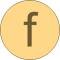# find

Find element in a vector# Information

This information is part of the Modelica Standard Library maintained by the Modelica Association.

#### Syntax

```Vectors.find(e, v);
Vectors.find(e, v, eps=0);
```

#### Description

The function call "`Vectors.find(e, v)`" returns the index of the first occurrence of input e in vector v. The test of equality is performed by "abs(e-v[i]) ≤ eps", where "eps" can be provided as third argument of the function. Default is "eps = 0".

#### Example

```  Real v = {1, 2, 3};
Real e1 = 2;
Real e2 = 3.01;
Boolean result;
algorithm
result := Vectors.find(e1,v);          // = 2
result := Vectors.find(e2,v);          // = 0
result := Vectors.find(e2,v,eps=0.1);  // = 3
```